(Authors: LE Bantis, B Brewer, CT Nakas, B Reiser)

This package is focused on Box-Cox based ROC curves and provides point estimates, confidence intervals (CIs), and hypothesis tests. It can be used both for inferences for a single biomarker and when comparisons of two correlated biomarkers are of interest. It provides inferences and comparisons around the area under the ROC curve (AUC), the Youden index, the sensitivity at a given specificity level (and vice versa), the optimal operating point of the ROC curve (in the Youden sense), and the Youden based cutoff. This documentation consists of two parts, the one marker and the two marker case. All approaches presented herein have been recently published (see references for each function).

The functions of each part:

• One marker case

• checkboxcox
• rocboxcox
• rocboxcoxCI
• Two marker case (comparisons of two markers)

• comparebcAUC
• comparebcJ
• comparebcSens
• comparebcSpec

checkboxcox

• Description

• This function tests whether the Box-Cox transformation is able to achieve approximate normality for your data. That is, it will allow the user to know whether it is appropriate to use all the methods discussed later on in this package.
• Usage
checkboxcox(marker, D, plots, printShapiro = TRUE)

• Arguments

• marker: It is a vector of length $$n$$ that contains the biomarker scores of all individuals.
• D: It is a vector of length $$n$$ that contains the true disease status of an individual. It is a binary vector containing 0 for the healthy/control individuals and 1 for the diseased individuals.
• plots='on' or 'off': When set to 'on', the user gets the histograms of the biomarker for both the healthy and the diseased group before and after the Box-Cox transformation. In addition, all four corresponding qq-plots are provided. When set to 'off', these plots are suppressed.
• printShapiro: Boolean. When set to TRUE, the results of the Shapiro-Wilk test will be printed to the console. When set to FALSE, the results are suppressed. Default value is FALSE.
• Value

• res_shapiro: A results table that contains the results of four Shapiro-Wilk tests for normality testing. Two of these refer to normality testing of the healthy and the diseased groups before the Box-Cox transformation, and the remaining two refer to the Box-Cox transformed biomarkers scores for the healthy and the diseased groups. Thus, this testing process produces four p-values. In addition, if the plots are set to 'on', then the output provides (1) the histograms of the biomarker for both the healthy and the diseased groups before and after the Box-Cox transformation, (2) all four corresponding qq-plots, and (3) a plot with the empirical ROC curve overplotted with the Box-Cox based ROC curve for visual comparison purposes.
• transformation.parameter: The single transformation parameter $$\lambda$$ that is applied for both groups simultaneously.
• transx: The Box-Cox transformed scores for the healthy.
• transy: The Box-Cox transformed scores for the diseased.
• pval_x: The p-value of the Shapiro-Wilk test of normality for the healthy group (before the Box-Cox transformation).
• pval_y: The p-value of the Shapiro-Wilk test of normality for the diseased group (before the Box-Cox transformation).
• pval_transx: The p-value of the Shapiro-Wilk test of normality for the healthy group (after the Box-Cox transformation).
• pval_transy: The p-value of the Shapiro-Wilk test of normality for the diseased group (after the Box-Cox transformation).
• *Example: *

#DATA GENERATION
set.seed(123)
x=rgamma(100, shape=2, rate = 8) # Generates biomarker data from a gamma distribution
# for the healthy group.
y=rgamma(100, shape=2, rate = 4) # Generates biomarker data from a gamma distribution
# for the diseased group.
scores=c(x,y)
D=c(zeros(1,100), ones(1,100))

out=checkboxcox(marker=scores, D, plots="on", printShapiro = TRUE)

##
##  Shapiro-Wilk normality test
##
## data:  x
## W = 0.92354, p-value = 2.181e-05
##
##
##  Shapiro-Wilk normality test
##
## data:  y
## W = 0.90169, p-value = 1.7e-06
##
##
##  Shapiro-Wilk normality test
##
## data:  transx
## W = 0.98765, p-value = 0.4826
##
##
##  Shapiro-Wilk normality test
##
## data:  transy
## W = 0.98936, p-value = 0.6128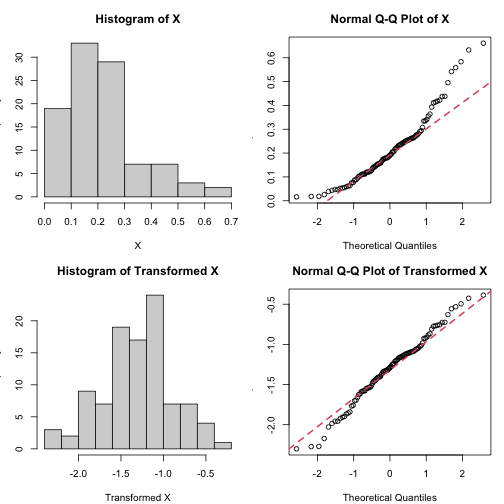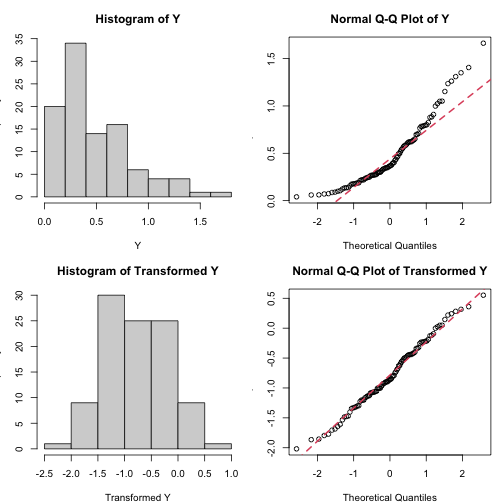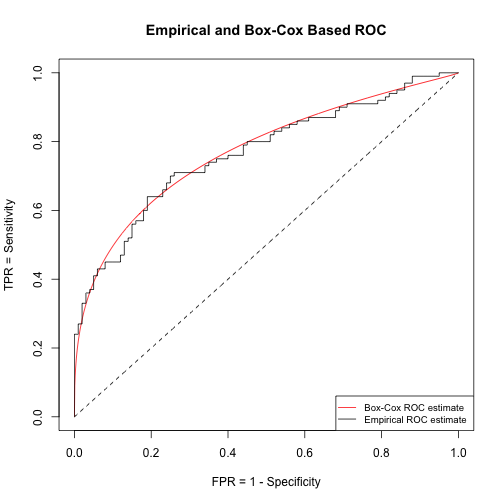summary(out)

##                          Length Class  Mode
## transformation.parameter   1    -none- numeric
## transx                   100    -none- numeric
## transy                   100    -none- numeric
## pval_x                     1    -none- numeric
## pval_y                     1    -none- numeric
## pval_transx                1    -none- numeric
## pval_transy                1    -none- numeric
## rocbc                      1    -none- function


rocboxcox

• Description

• This function applies the Box-Cox transformation to provide a comprehensive ROC analysis that involves the AUC (and its CI), the maximized Youden index (and its CI), the optimized Youden based cutoff (and its CI), and joint confidence regions for the optimized pair of sensitivity and specificity. For the AUC and the Youden index, the Delta Method is employed by accounting for the variability of the estimated transformation parameters due to the Box-Cox transformation. Both the AUC and the YI confidence intervals are found after using the probit transformation and back-transforming the endpoints of the corresponding CI back into the ROC space. For the cutoffs, it has been shown that the delta method does not perform well; thus, the bootstrap with 1,000 repetitions is employed here, instead. During this this process, the cutoffs are back-transformed with the inverse Box-Cox transformation so that they lie on the original scale of the data.
• Usage
rocboxcox(marker, D, plots, printProgress = TRUE)

• Arguments

• marker: It is a vector of length $$n$$ that contains the biomarker scores of all individuals.
• D: It is a vector of length $$n$$ that contains the true disease status of an individual. It is a binary vector containing 0 for the healthy/control individuals and 1 for the diseased individuals.
• plots='on' or 'off': When set to 'on', it returns a comprehensive figure with the ROC estimate and several point estimates: AUC, Youden index, optimized Youden cutoff, Youden based Sens and Spec along with the corresponding marginal confidence intervals and the joint confidence region of the estimated Sens and Spec. When set to 'off', these plots and corresponding information tidbits are suppressed.
• printProgress: Boolean. When set to TRUE, messages describing the progress of the bootstrapping will be printed to the console window. When set to FALSE, these messages are suppressed. Default value is FALSE.
• Value

• transx: The Box-Cox transformed scores for the healthy group.
• transy: The Box-Cox transformed scores for the diseased group.
• transformation.parameter: The estimated Box-Cox transformation parameter ($$\lambda$$).
• AUC: The estimated area under the Box-Cox based ROC curve.
• AUCCI: The (1-$$\alpha$$)100\% CI for the AUC. (A common choice of $$\alpha$$ is 0.05). This CI is based on probit transforming the AUC estimate, finding the CI on the real line, and then back-transforming its endpoints to the ROC space. It is in line with using $$Z=\frac{\Phi^{-1}(\hat{AUC})}{\sqrt{var(\Phi^{-1}(\hat{AUC}))}}$$ to test the null hypothesis that $$H_{0}: AUC=0.5$$.
• pvalueAUC: The corresponding p-value for the AUC estimate. This a two-tailed p-value that tests the hypothesis $$H_{0}: AUC=0.5$$ by employing $$Z=\frac{\Phi^{-1}(\hat{AUC})}{\sqrt{var(\Phi^{-1}(\hat{AUC}))}}$$ which, under the null hypothesis, is taken to follow a standard normal distribution.
• J: The maximized Youden index.
• JCI: The corresponding CI for the maximized Youden index. For this CI, we consider the probit transformation $$\hat{J}_{T}=\Phi^{-1}(\hat{J})$$ and then back-transform its endpoints to derive a 95\% CI for the Youden index itself (Bantis et al. (2021)).
• pvalueJ: the corresponding two-tailed p-value for the maximized Youden index. This corresponds to $$Z=\frac{\hat{J}_{T}}{\sqrt{var(\hat{J}_{T})}}$$. The underlying null hypothesis is $$H_{0}: J=0$$
• Sens: The sensitivity that corresponds to the Youden based optimized cutoff.
• CImarginalSens: The marginal (1-$$\alpha$$)100\% CI for the sensitivity that corresponds to the Youden based optimized cutoff. This is derived by first employing the probit transformation, finding a CI on the real line, and then back-transforming its endpoints to the ROC space.
• Spec: The specificity that corresponds to the Youden based optimized cutoff.
• CImarginalSpec: The marginal (1-$$\alpha$$)100\% CI for the specificity that corresponds to the Youden based optimized cutoff. This is derived by first employing the probit transformation, finding a CI on the real line, and then back-transforming its endpoints to the ROC space.
• cutoff: The Youden based optimized cutoff.
• CIcutoff: the (1-$$\alpha$$)100\% CI for the Youden-based optimized cutoff. This is based on the bootstrap. It involves using the Box-Cox transformation for every bootstrap iteration and then using the inverse Box-Cox transformation to obtain the cutoff on its original scale (Bantis et al. (2019)).
• areaegg: the area of the (1-$$\alpha$$)100\% egg-shaped joint confidence region that refers to the optimized pair of sensitivity and specificity. This takes into account the fact that the estimated sensitivity and specificity are correlated as opposed to the corresponding rectangular area that ignores this.
• arearect: the area of the (1-$$\alpha$$)100\% rectangular joint confidence region that refers to the optimized pair of sensitivity and specificity. This ignores the correlation of the optimized sensitivity and specificity and tends to yield a larger area compared to the one of the egg-shaped region.
• mxlam: The mean of the marker scores of the healthy group after the Box-Cox transformation.
• sxlam: The standard deviation of the marker scores of the healthy group after the Box-Cox transformation.
• mylam: The mean of the marker scores of the diseased group after the Box-Cox transformation.
• sylam: The standard deviation of the marker scores of the diseased group after the Box-Cox transformation.
• results: A table that provides some indicative results: the AUC, the J (maximized Youden index), the estimated cutoff, the Sens, and the Spec along with their marginal CIs.
• rocfun: A function of the estimated Box-Cox ROC curve. You can use this to simply request TPR values for given FPR values.
• *Example: *

set.seed(123)
x=rgamma(100, shape=2, rate = 8)
y=rgamma(100, shape=2, rate = 4)
scores=c(x,y)
D=c(zeros(1,100), ones(1,100))
out=rocboxcox(marker=scores,D, 0.05, plots="on", printProgress=FALSE)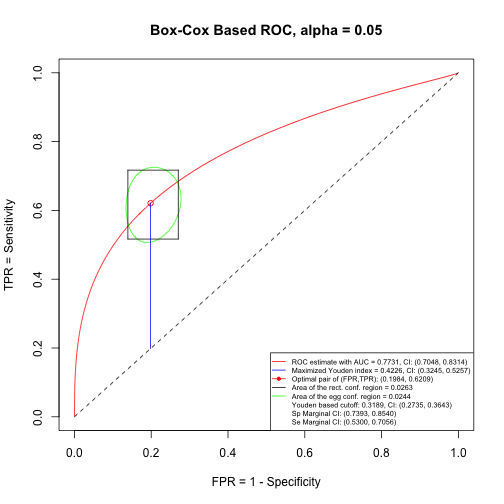rocboxcoxCI

• Description

• This function applies the Box-Cox transformation and carries out statistical inferences for the sensitivity at a given specificity (and vice versa).
• Usage
out=rocboxcoxCI(marker, D, givenSP=givenSP, givenSE=NA, alpha, plots)

out=rocboxcoxCI(marker, D, givenSP=NA, givenSE=givenSE, alpha, plots)

• Arguments

• marker: It is a vector of length $$n$$ that contains the biomarker scores of all individuals.
• D: It is a vector of length $$n$$ that contains the true disease status of an individual. It is a binary vector containing 0 for the healthy/control individuals and 1 for the diseased individuals.
• givenSP: It is a vector of specificity values that the user wants to fix/set, at which the sensitivity is to be estimated. In this case, the 'givenSE' argument needs to be set to NA.
• givenSE: It is a vector of sensitivity values that the user want to fix/set, at which the specificity is to be estimated. In this case, the 'givenSE' argument needs to be set to NA.
• alpha: Nominal level used to calculate the confidence intervals. A common choice is 0.05.
• plots='on' or 'off': When set to 'on', it returns the Box-Cox based ROC plot along with pointwise 95\% confidence intervals for the full spectrum of FPRs. A second plot is also provided that visualizes the confidence intervals at the given sensitivities or specificities. When set to 'off', both plots are suppressed.
• Value

• SPandCIs: The specificity values and the CIs around them.
• SEandCIs: The specificity values and the CIs around them.
• SEvalues: The sensitivity values provided by the user at which the specificity was calculated. If the user did not provide any sensitivity values, this argument is set to NA.
• SPvalues: tTe specificity values provided by the user at which the sensitivity was calculated. If the user did not provide any specificity values, this argument is set to NA.
• *Example: *

givenSP=c(0.1,0.2,0.3,0.4,0.5,0.6,0.7,0.8,0.9)
givenSE=NA
out=rocboxcoxCI(marker=scores ,D, givenSP=givenSP, givenSE=NA, alpha=0.05, plots="on")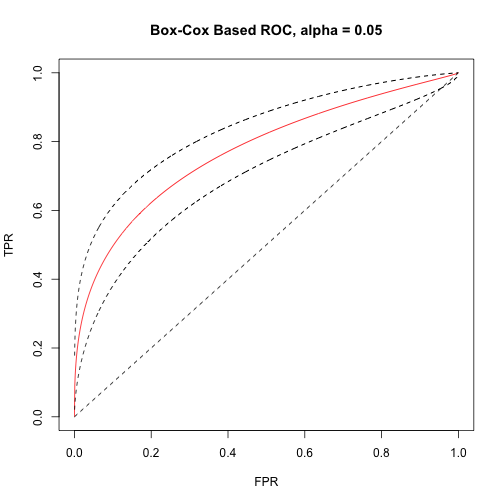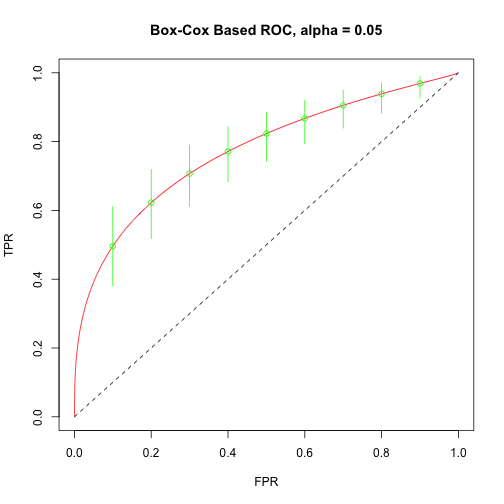#


comparebcAUC

• Description

• This function provides a comparison of two correlated markers in terms of their AUCs (areas under the Box-Cox based ROC curves). Marker measurements are assumed to be taken on the same individuals for both markers.
• Usage
out=comparebcAUC(marker1, marker2, D, alpha, plots)

• Arguments

• marker1: It is a vector of length $$n$$ that contains the biomarker scores of all individuals for the first marker.
• marker2: It is a vector of length $$n$$ that contains the biomarker scores of all individuals for the second marker.
• D: It is a binary vector of length $$n$$ that contains the true disease status of an individual. It is a binary vector containing 0 for the healthy/control individuals and 1 for the diseased individuals.
• alpha: Nominal level used to calculate the confidence intervals. A common choice is 0.05.
• plots='on' or 'off': When set to 'on', it returns the Box-Cox based ROC along with informative information about the two AUCs in the legend of the plot. When set to 'off', these plots are suppressed.
• Value

• AUCmarker1: the AUC of the first marker
• AUCmarker2: the AUC of the second marker
• pvalue_probit_difference: the p-value for the comparison of the AUCs. It employs the probit transformation that has greater power then when not using it (as opposed to the p-value provided in the 'pvalue difference' output argument provided later on). It is based on $$Z^*=\frac{\Phi^{-1}\left(\hat{AUC}_{1}\right)-\Phi^{-1}\left(\hat{AUC}_{2}\right)}{\sqrt{Var\left(\Phi^{-1}\left(\hat{AUC}_{1}\right)\right)+Var\left(\Phi^{-1}\left(\hat{AUC}_{2}\right)\right)-2Cov\left(\Phi^{-1}\left(\hat{AUC}_{1}\right)),\Phi^{-1}\left(\hat{AUC}_{2}\right)\right)}}$$.
• CI_probit_difference: the confidence interval for the difference of the probits of the AUCs. (corresponds to the same strategy as the 'pvalue_probit_difference'). It is also based on $$Z^*$$ given above.
• pvalue_difference: the p-value for the comparisons of the AUC (without the probit transformation). Simulations have shown that this is inferior to the 'pvalue probit difference'. This is based on $$Z=\frac{\hat{AUC}_{1}-\hat{AUC}_{2}}{\sqrt{Var\left(\hat{AUC}_{1}\right)+Var\left(\hat{AUC}_{2}\right)-2Cov\left(\hat{AUC}_{1},\hat{AUC}_{2}\right)}}$$.
• CI_difference: the confidence interval for the difference of the AUCs. It is based on $$Z$$ given above.
• roc1: a function that refers to the ROC of the first marker. It allows the user to feed in FPR values and the corresponding TPR values.
• roc2: a function that refers to the ROC of the first marker. It allows the user to feed in FPR values and the corresponding TPR values.
• transx1: the Box-Cox transformed scores for the first marker and the healthy group.
• transy1: the Box-Cox transformed scores for the first marker and the diseased group.
• transx2: the Box-Cox transformed scores for the second marker and the healthy group.
• transy2: the Box-Cox transformed scores for the second marker and the diseased group.
• *Example: *

#GENERATE SOME BIVARIATE DATA===
set.seed(123)

nx <- 100
Sx <- matrix(c(1,   0.5,
0.5,  1),
nrow = 2, ncol = 2)

mux <- c(X = 10, Y = 12)
X=rmvnorm(nx, mean = mux, sigma = Sx)

ny <- 100
Sy <- matrix(c(1.1,   0.6,
0.6,  1.1),
nrow = 2, ncol = 2)

muy <- c(X = 11, Y = 13.7)
Y=rmvnorm(ny, mean = muy, sigma = Sy)

dx=zeros(nx,1)
dy=ones(ny,1)

markers=rbind(X,Y);
marker1=markers[,1]
marker2=markers[,2]
D=c(rbind(dx,dy))

#==DATA HAVE BEEN GENERATED====

#===COMPARE THE AUCs of Marker 1 vs Maker 2

out=comparebcAUC(marker1, marker2, D, alpha=0.05,  plots="on")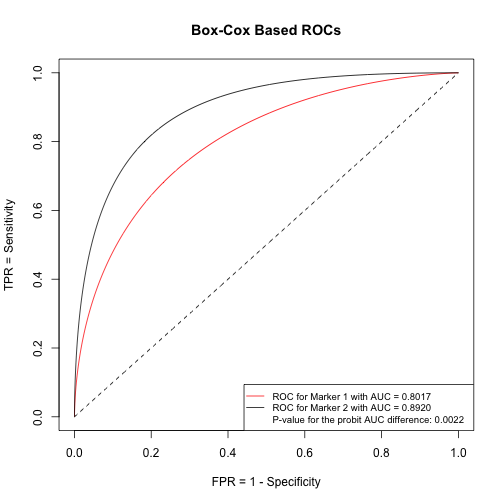comparebcJ

• Description

• This function provides a comparison of two correlated markers in terms of their J (Youden indices for Box-Cox based ROC curves). Markers measurements are assumed to be taken on the same individuals for both markers.
• Usage
out=comparebcJ(marker1, marker2, D, alpha, plots)

• Arguments

• marker1: It is a vector of length $$n$$ that contains the biomarker scores of all individuals for the first marker.
• marker2: It is a vector of length $$n$$ that contains the biomarker scores of all individuals for the second marker.
• D: It is a vector of length $$n$$ that contains the true disease status of an individual. It is a binary vector containing 0 for the healthy/control individuals and 1 for the diseased individuals.
• alpha: Nominal level based on which the confidence intervals are going to be calculated. A common choice is 0.05.
• plots='on' or 'off': When set to 'on', it returns the Box-Cox based ROC along with informative information about the two AUCs in the legend of the plot.
• Value

• J1: The maximized Youden index (J) of the first marker.
• J2: The maximized Youden index (J) of the second marker.
• pvalue_probit_difference: The p-value for the comparison of the Js. It employs the probit transformation that has greater power then when not using it (as opposed to the p-value provided in the 'pvalue difference' output argument provided later on). It is based on $$Z^{*}=\frac{\hat{J}_{T2}-\hat{J}_{T1}}{\sqrt{Var(\hat{J}_{T1})+Var(\hat{J}_{T2})-2Cov(\hat{J}_{T1},\hat{J}_{T2})}}$$ where $$\hat{J}_{Ti}=\Phi^{-1}(\hat{J}_{i})$$, $$i=1,2$$.
• CI_probit_difference: The confidence interval for the difference of the transformed Js. (Corresponds to the same strategy as the 'pvalue_trans_difference' and is based on $$Z^*$$ mentioned above).
• pvalue_difference: The p-value for the comparisons of the J (without the probit transformation). Simulations have shown that this is inferior to the 'pvalue probit difference'. This is based on $$Z=\frac{\hat{J}_{2}-\hat{J}_{1}}{\sqrt{Var(\hat{J}_{1})+Var(\hat{J}_{2})-2Cov(\hat{J}_{1},\hat{J}_{T})}}$$.
• CI_difference: The confidence interval for the difference of the Js. This is based on $$Z$$ mentioned right above.
• roc1: A function that refers to the ROC of the first marker. It allows the user to feed in FPR values and the corresponding TPR values.
• roc2: A function that refers to the ROC of the first marker. It allows the user to feed in FPR values and the corresponding TPR values.
• transx1: The Box-Cox transformed scores for the first marker and the healthy group.
• transy1: The Box-Cox transformed scores for the first marker and the diseased group.
• transx2: The Box-Cox transformed scores for the second marker and the healthy group.
• transy2: The Box-Cox transformed scores for the second marker and the diseased group.
• *Example: *

#==DATA HAVE BEEN GENERATED====
#===COMPARE THE Js of Marker 1 vs Maker 2

out=comparebcJ(marker1, marker2, D, alpha=0.05,  plots="on")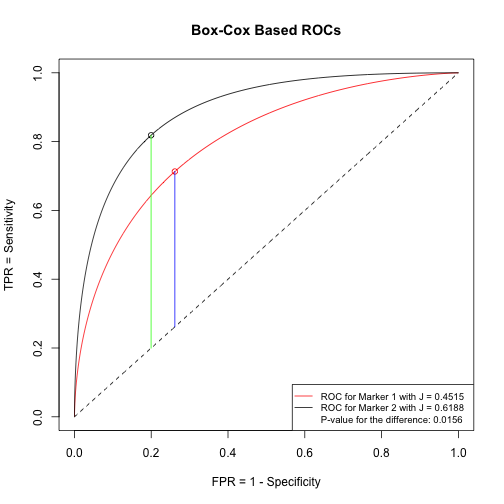#


comparebcSens

• Description

• This function provides a comparison of two correlated markers in terms of their sensitivities at a given specificity (for Box-Cox based ROC curves). Marker measurements are assumed to be taken on the same individuals for both markers.
• Usage
out=comparebcSens(marker1, marker2, D, alpha, atSpec, plots)

• Arguments

• marker1: It is a vector of length $$n$$ that contains the biomarker scores of all individuals for the first marker.
• marker2: It is a vector of length $$n$$ that contains the biomarker scores of all individuals for the second marker.
• D: It is a vector of length $$n$$ that contains the true disease status of an individual. It is a binary vector containing 0 for the healthy/control individuals and 1 for the diseased individuals.
• alpha: Nominal level used to calculate the confidence intervals. A common choice is 0.05.
• atSpec: The value of specificity at which the comparison of sensitivities will take place (a single value / scalar).
• plots='on' or 'off': When set to 'on', it returns the Box-Cox based ROC along with informative information about the two AUCs in the legend of the plot. When set to 'off', these plots are suppressed.
• Value

• Sens1: The sensitivity at the selected specificity for the first marker.
• Sens2: The sensitivity at the selected specificity for the second marker.
• pvalue_probit_difference: The p-value for the comparison of the sensitivities. It employs the probit transformation that has greater power then when not using it (as opposed to the p-value provided in the 'pvalue_difference' output argument provided later on).
• CI_probit_difference: The confidence interval for the difference of the probits of the sensitivities. (corresponds to the same strategy as the 'pvalue_probit_difference').
• pvalue_difference: The p-value for the comparisons of the sensitivities (without the probit transformation). Simulations have shown that this is inferior to the 'pvalue_probit_difference'.
• CI_difference: The confidence interval for the difference of the sensitivities.
• roc1: A function that refers to the ROC of the first marker. It allows the user to feed in FPR values and the corresponding TPR values.
• roc2: A function that refers to the ROC of the first marker. It allows the user to feed in FPR values and the corresponding TPR values.
• transx1: The Box-Cox transformed scores for the first marker and the healthy group.
• transy1: The Box-Cox transformed scores for the first marker and the diseased group.
• transx2: The Box-Cox transformed scores for the second marker and the healthy group.
• transy2: The Box-Cox transformed scores for the second marker and the diseased group.
• *Example: *

out=comparebcSens(marker1=marker1, marker2=marker2, D=D, alpha =0.05, atSpec=0.8, plots="on")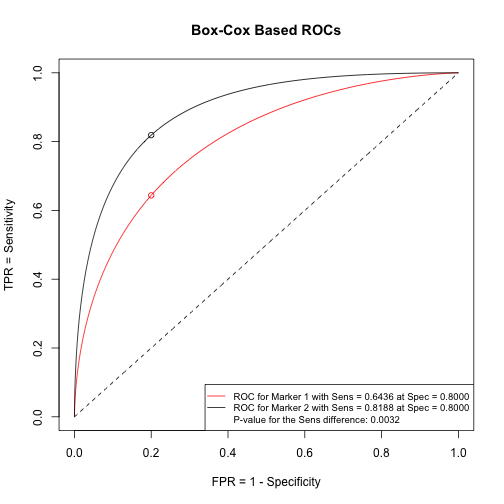summary(out)

##                          Length Class       Mode
## resultstable               6    formattable numeric
## Sens1                      1    -none-      numeric
## Sens2                      1    -none-      numeric
## pvalue_probit_difference   1    -none-      numeric
## pvalue_difference          1    -none-      numeric
## CI_difference              2    -none-      numeric
## roc1                       1    -none-      function
## roc2                       1    -none-      function
## transx1                  100    -none-      numeric
## transy1                  100    -none-      numeric
## transx2                  100    -none-      numeric
## transy2                  100    -none-      numeric

#


comparebcSpec

• Description

• This function provides a comparison of two correlated markers in terms of their specificities at a given sensitivity (for Box-Cox based ROC curves). Marker measurements are assumed to be taken on the same individuals for both markers.
• Usage
out=comparebcSpec(marker1, marker2, D, alpha, atSens, plots)

• Arguments

• marker1: It is a vector of length $$n$$ that contains the biomarker scores of all individuals for the first marker.
• marker2: It is a vector of length $$n$$ that contains the biomarker scores of all individuals for the second marker.
• D: It is a vector of length $$n$$ that contains the true disease status of an individual. It is a binary vector containing 0 for the healthy/control individuals and 1 for the diseased individuals.
• alpha: Nominal level used to calculate the confidence intervals. A common choice is 0.05.
• atSens: The value of sensitivity at which the comparison of specificities will take place (a single value / scalar).
• plots='on' or 'off': When set to 'on' it returns the Box-Cox based ROC along with informative information about the two AUCs in the legend of the plot. When set to 'off', this information is suppressed.
• Value

• Spec1: The specificity at the selected sensitivity for the first marker.
• Spec2: The specificity at the selected sensitivity for the second marker.
• pvalue_probit_difference: The p-value for the comparison of the specificities. It employs the probit transformation that has greater power then when not using it (as opposed to the p-value provided in the 'pvalue_difference' output argument provided later on).
• CI_probit_difference: The confidence interval for the difference of the probits of the specificities (corresponds to the same strategy as the 'pvalue_probit_difference').
• pvalue_difference: The p-value for the comparison of the specificities (without the probit transformation). Simulations have shown that this is inferior to the 'pvalue_probit_difference'.
• CI_difference: The confidence interval for the difference of the specificities.
• roc1: A function that refers to the ROC of the first marker. It allows the user to feed in FPR values and the corresponding TPR values.
• roc2: A function that refers to the ROC of the first marker. It allows the user to feed in FPR values and the corresponding TPR values.
• transx1: The Box-Cox transformed scores for the first marker and the healthy group.
• transy1: The Box-Cox transformed scores for the first marker and the diseased group.
• transx2: The Box-Cox transformed scores for the second marker and the healthy group.
• transy2: The Box-Cox transformed scores for the second marker and the diseased group.
• *Example: *

out=comparebcSpec(marker1=marker1, marker2=marker2, D=D, alpha =0.05, atSens=0.8, plots="on")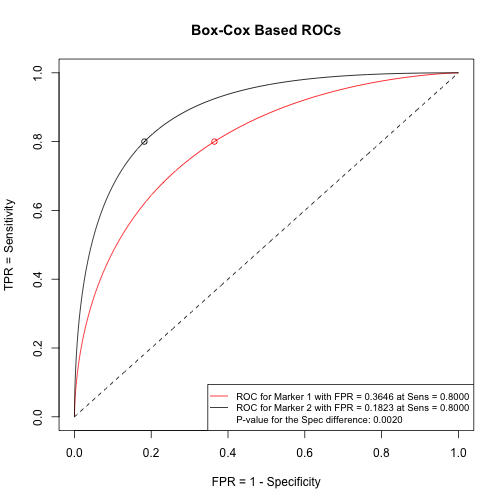summary(out)

##                          Length Class       Mode
## resultstable               6    formattable numeric
## FPR1                       1    -none-      numeric
## FPR2                       1    -none-      numeric
## pvalue_probit_difference   1    -none-      numeric
## pvalue_difference          1    -none-      numeric
## CI_difference              2    -none-      numeric
## roc1                       1    -none-      function
## roc2                       1    -none-      function
## transx1                  100    -none-      numeric
## transy1                  100    -none-      numeric
## transx2                  100    -none-      numeric
## transy2                  100    -none-      numeric

#


The package is available at https://www.leobantis.net and soon to be submitted to CRAN.